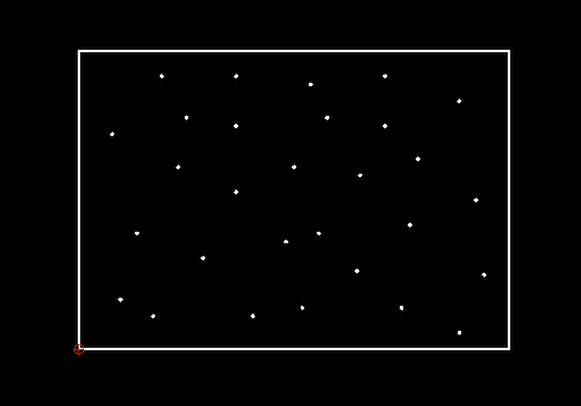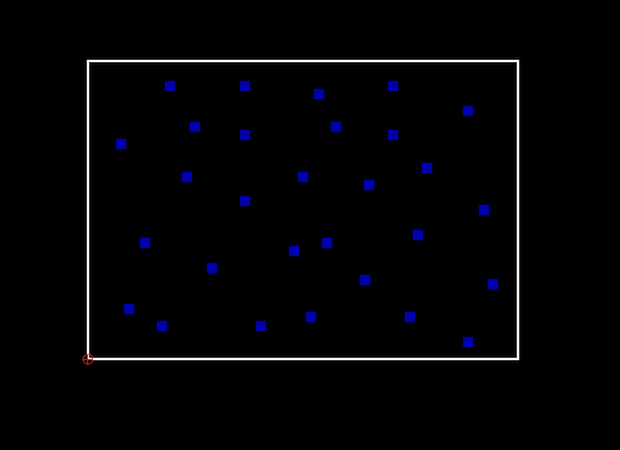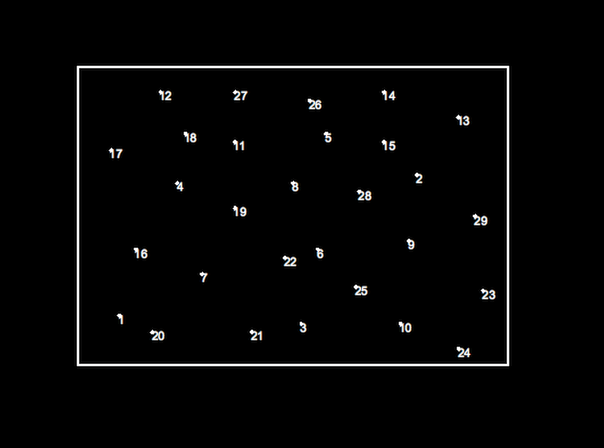# Tutorial: Reusing Drawing Positions

## Introduction

There are situations in which existing positions of a drawing are to be used as basis for a new operation. The positions may for example be defined as points or other entities at those positions. The positions are not in any regular shape or pattern but arbitrary coordinates, perhaps as measured from an existing situation.

## Example

For the sake of this example, let's assume the points shown in this example are such positions and we want to add a unique number to each point.For this example, we add a text label with a counter at each position of a point. However, we could also add a circle around each point or paste the clipboard at each location of a point.

Rather than clicking each point individually, QCAD can record (store) those positions and then replay or reuse them for another tool. This can be any tool that expects coordinates as input, for example a tool for drawing points, lines or circles with given radius, pasting the clipboard, adding text, inserting a block, etc.

## Step 1: Selecting the Positions

First, select all the entities that define the positions you want to work with. Here, we select the points:## Step 2: Storing the Positions

Choose Misc > Information > Store Positions to store the positions of the selected entities. Positions are stored in drawing / display order.

For points, the coordinate of the point is stored. For lines, the start point of each line. For polyline, each vertex. For splines, each fit point or control point.

## Step 3: Starting the Tool to Use the Positions

Next, start the tool you wish to use the positions for. In this example, we add a counter to each point in drawing order using Misc > Draw > Counter.

## Step 4: Using the Stored Positions

Instead of clicking each point now, we can simply choose Misc > Information > Use Positions and QCAD "clicks" each stored position for us:## Other Example Uses

• Refactoring drawings with many repeated loose patterns of entities to use block references.
For example, replacing all identical circles with a block reference of a block containing a circle. All circles can then be changed at once by changing that block.
• Pasting entities at each vertex of a polyline.
• Drawing a point entity at each control point of a spline.
• Adding counters to entities / vertices.
• Creating polylines based on points or circle centers.
• Replacing points with circles.
• etc.